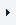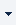LOGINREGISTER (FREE TRIAL)myTOMLAB
 ProductsTOMLAB Base ModuleTOMLAB /MINOSTOMLAB /NPSOLTOMLAB /DNOPTTOMLAB /SNOPTTOMLAB /SOLTOMLAB /CGOTOMLAB /CPLEXTOMLAB /GUROBITOMLAB /MINLPSolvers bqpd filterSQP minlpBB miqpBBTOMLAB /MIPNLPTOMLAB /MISQPTOMLAB /PENSDPTOMLAB /PENBMITOMLAB /KNITROTOMLAB /OQNLPTOMLAB /CONOPTTOMLAB /PROPTTOMLAB /NLPQLTOMLAB /LGOTOMLAB /LGO-MINLPTOMLAB /GPTOMLAB /GENOTOMLAB /MADTOMLAB /MIDACO

# bqpd

The solver BQPD solves large, sparse or dense linear and quadratic programming problems.

## Main features

• The BQPD code solves quadratic programming (minimization of a quadratic function subject to linear constraints) and linear programming problems. If the Hessian matrix Q is positive definite, then a global solution is found. A global solution is also found in the case of linear programming (Q=0). When Q is indefinite, a Kuhn-Tucker point that is usually a local solution is found.

• The code implements a null-space active set method with a technique for resolving degeneracy that guarantees that cycling does not occur even when round-off errors are present. Feasibility is obtained by minimizing a sum of constraint violations. The Devex method for avoiding near-zero pivots is used to promote stability. The matrix algebra is implemented so that the algorithm can take advantage of sparse factors of the basis matrix. Factors of the reduced Hessian matrix are stored in a dense format, an approach that is most effective when the number of free variables is relatively small. The user must supply a subroutine to evaluate the Hessian matrix Q, so that sparsity in Q can be exploited. An extreme case occurs when Q=0 and the QP reduces to a linear program. The code is written to take maximum advantage of this situation, so that it also provides an efficient method for linear programming.

• The dense and the sparse version of BQPD are compiled in two different MEX binaries, making the two versions optimally efficient.

• BQPD is integrated with the TOMLAB driver routines.

• BQPD may be used as subproblem solver in the TOMLAB environment.